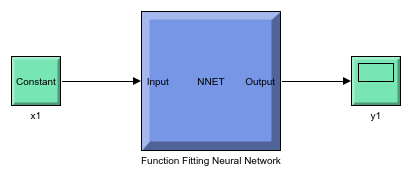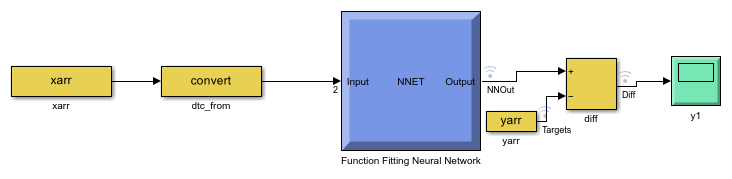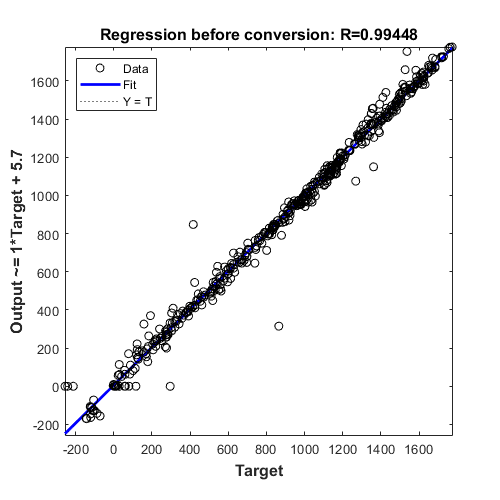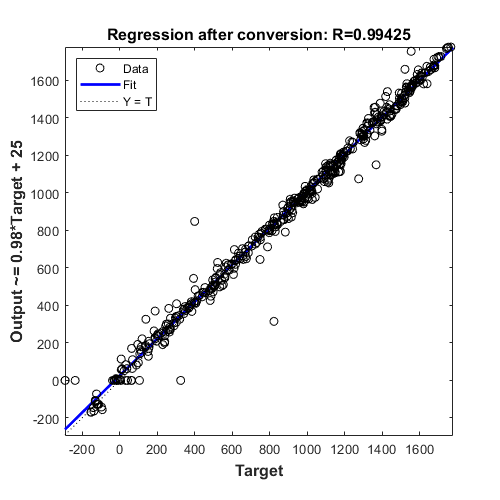# Convert Neural Network Algorithms to Fixed-Point using fxpopt and Generate HDL Code

This example shows how to convert a neural network regression model in Simulink to fixed point using the `fxpopt` function and Lookup Table Optimizer.

### Overview

Fixed-Point Designer provides workflows via the Fixed Point Tool that can convert a design from floating-point data types to fixed-point data types. The `fxpopt` function optimizes data types in a model based on specified system behavioral constraints. For additional information, refer to the documentation link https://www.mathworks.com/help/fixedpoint/ref/fxpopt.html The Lookup Table Optimizer generates memory-efficient lookup table replacements for unbounded functions such as `exp` and `log2`. Using these tools, this example showcases how to convert a trained floating-point neural network regression model to use embedded-efficient fixed-point data types.

### Data and Neural Network Training

The `engine_dataset` contains data representing the relationship between the fuel rate and speed of the engine, and its torque and gas emissions.

```% Use the function fitting tool (nftool) from Deep Learning Toolbox (TM) to % train a neural network to estimate torque and gas emissions of an engine % given the fuel rate and speed. Use the following commands to train % the neural network. load engine_dataset; x = engineInputs; t = engineTargets; net = fitnet(10); net = train(net,x,t); view(net) ```Close all windows of training tool and view of the network.

```nnet.guis.closeAllViews(); nntraintool('close'); ```

### Model Preparation for Fixed-Point Conversion

Once the network is trained, use the `gensim` function from the Deep Learning Toolbox (TM) to generate a Simulink model.

```[sysName, netName] = gensim(net, 'Name', 'mTrainedNN'); ```The model generated by the `gensim` function contains the neural network with trained weights and biases. To prepare this generated model for fixed-point conversion, follow the preparation steps in the best practices guidelines. https://www.mathworks.com/help/fixedpoint/ug/best-practices-for-using-the-fixed-point-tool-to-propose-data-types-for-your-simulink-model.html

After applying these principles, the trained neural network is further modified to enable signal logging at the output of the network, add input stimuli and verification blocks.

Open and inspect the model.

```model = 'ex_fxpdemo_neuralnet_regression'; system_under_design = [model '/Function Fitting Neural Network']; baseline_output = [model '/yarr']; open_system(model); % Set up model for HDL code generation hdlsetup(model); ```
```### <a href="matlab:configset.internal.open('ex_fxpdemo_neuralnet_regression','SingleTaskRateTransMsg')">SingleTaskRateTransMsg</a> value is set from 'none' to 'error' (<a href="matlab:set_param('ex_fxpdemo_neuralnet_regression','SingleTaskRateTransMsg', 'none')">revert</a>). ### <a href="matlab:configset.internal.open('ex_fxpdemo_neuralnet_regression','Solver')">Solver</a> value is set from 'FixedStepAuto' to 'FixedStepDiscrete' (<a href="matlab:set_param('ex_fxpdemo_neuralnet_regression','Solver', 'FixedStepAuto')">revert</a>). ### <a href="matlab:configset.internal.open('ex_fxpdemo_neuralnet_regression','AlgebraicLoopMsg')">AlgebraicLoopMsg</a> value is set from 'warning' to 'error' (<a href="matlab:set_param('ex_fxpdemo_neuralnet_regression','AlgebraicLoopMsg', 'warning')">revert</a>). ### <a href="matlab:configset.internal.open('ex_fxpdemo_neuralnet_regression','BlockReduction')">BlockReduction</a> value is set from 'on' to 'off' (<a href="matlab:set_param('ex_fxpdemo_neuralnet_regression','BlockReduction', 'on')">revert</a>). ### <a href="matlab:configset.internal.open('ex_fxpdemo_neuralnet_regression','ConditionallyExecuteInputs')">ConditionallyExecuteInputs</a> value is set from 'on' to 'off' (<a href="matlab:set_param('ex_fxpdemo_neuralnet_regression','ConditionallyExecuteInputs', 'on')">revert</a>). ### <a href="matlab:configset.internal.open('ex_fxpdemo_neuralnet_regression','DefaultParameterBehavior')">DefaultParameterBehavior</a> value is set from 'Tunable' to 'Inlined' (<a href="matlab:set_param('ex_fxpdemo_neuralnet_regression','DefaultParameterBehavior', 'Tunable')">revert</a>). ### <a href="matlab:configset.internal.open('ex_fxpdemo_neuralnet_regression','ProdHWDeviceType')">ProdHWDeviceType</a> value is set from 'Intel->x86-64 (Windows64)' to 'ASIC/FPGA->ASIC/FPGA' (<a href="matlab:set_param('ex_fxpdemo_neuralnet_regression','ProdHWDeviceType', 'Intel->x86-64 (Windows64)')">revert</a>). ### The listed configuration parameter values are modified as a part of hdlsetup. Please refer to <a href="matlab:helpview(fullfile(docroot, 'hdlcoder', 'helptargets.map'), 'msg_hdlsetup_function')">hdlsetup</a> document for best practices on model settings. ```Simulate the model to observe model performance when using double-precision floating-point data types.

```loggingInfo = get_param(model, 'DataLoggingOverride'); sim_out = sim(model, 'SaveFormat', 'Dataset'); plotRegression(sim_out, baseline_output, system_under_design, 'Regression before conversion'); ```### Define System Behavioral Constraints for Fixed Point Conversion

```opts = fxpOptimizationOptions(); opts.addTolerance(system_under_design, 1, 'RelTol', 0.05); opts.addTolerance(system_under_design, 1, 'AbsTol', 50) opts.AllowableWordLengths = 8:32; ```

### Optimize Data Types

Use the `fxpopt` function to optimize the data types in the system under design and explore the solution. The software analyzes the range of objects in `system_under_design` and wordlength and tolerance constraints specified in `opts` to apply heterogeneous data types to the model while minimizing total bit width.

```solution = fxpopt(model, system_under_design, opts); best_solution = solution.explore; ```
``` + Checking for unsupported constructs. - The paths below have constructs that do not support fixed-point data types. These constructs will be surrounded with Data Type Conversion blocks. 'ex_fxpdemo_neuralnet_regression/Function Fitting Neural Network/Layer 1/tansig/tanh' + Preprocessing + Modeling the optimization problem - Constructing decision variables + Running the optimization solver - Evaluating new solution: cost 515, does not meet the tolerances. - Evaluating new solution: cost 577, does not meet the tolerances. - Evaluating new solution: cost 639, does not meet the tolerances. - Evaluating new solution: cost 701, does not meet the tolerances. - Evaluating new solution: cost 763, does not meet the tolerances. - Evaluating new solution: cost 825, does not meet the tolerances. - Evaluating new solution: cost 887, does not meet the tolerances. - Evaluating new solution: cost 949, meets the tolerances. - Updated best found solution, cost: 949 - Evaluating new solution: cost 945, meets the tolerances. - Updated best found solution, cost: 945 - Evaluating new solution: cost 944, meets the tolerances. - Updated best found solution, cost: 944 - Evaluating new solution: cost 943, meets the tolerances. - Updated best found solution, cost: 943 - Evaluating new solution: cost 942, meets the tolerances. - Updated best found solution, cost: 942 - Evaluating new solution: cost 941, meets the tolerances. - Updated best found solution, cost: 941 - Evaluating new solution: cost 940, meets the tolerances. - Updated best found solution, cost: 940 - Evaluating new solution: cost 939, meets the tolerances. - Updated best found solution, cost: 939 - Evaluating new solution: cost 938, meets the tolerances. - Updated best found solution, cost: 938 - Evaluating new solution: cost 937, meets the tolerances. - Updated best found solution, cost: 937 - Evaluating new solution: cost 936, meets the tolerances. - Updated best found solution, cost: 936 - Evaluating new solution: cost 926, meets the tolerances. - Updated best found solution, cost: 926 - Evaluating new solution: cost 925, meets the tolerances. - Updated best found solution, cost: 925 - Evaluating new solution: cost 924, meets the tolerances. - Updated best found solution, cost: 924 - Evaluating new solution: cost 923, meets the tolerances. - Updated best found solution, cost: 923 - Evaluating new solution: cost 922, meets the tolerances. - Updated best found solution, cost: 922 - Evaluating new solution: cost 917, meets the tolerances. - Updated best found solution, cost: 917 - Evaluating new solution: cost 916, meets the tolerances. - Updated best found solution, cost: 916 - Evaluating new solution: cost 914, meets the tolerances. - Updated best found solution, cost: 914 - Evaluating new solution: cost 909, meets the tolerances. - Updated best found solution, cost: 909 - Evaluating new solution: cost 908, meets the tolerances. - Updated best found solution, cost: 908 - Evaluating new solution: cost 906, meets the tolerances. - Updated best found solution, cost: 906 - Evaluating new solution: cost 898, meets the tolerances. - Updated best found solution, cost: 898 - Evaluating new solution: cost 897, meets the tolerances. - Updated best found solution, cost: 897 - Evaluating new solution: cost 893, does not meet the tolerances. - Evaluating new solution: cost 896, meets the tolerances. - Updated best found solution, cost: 896 - Evaluating new solution: cost 895, meets the tolerances. - Updated best found solution, cost: 895 - Evaluating new solution: cost 894, meets the tolerances. - Updated best found solution, cost: 894 - Evaluating new solution: cost 893, meets the tolerances. - Updated best found solution, cost: 893 - Evaluating new solution: cost 892, meets the tolerances. - Updated best found solution, cost: 892 - Evaluating new solution: cost 891, meets the tolerances. - Updated best found solution, cost: 891 - Evaluating new solution: cost 890, meets the tolerances. - Updated best found solution, cost: 890 - Evaluating new solution: cost 889, meets the tolerances. - Updated best found solution, cost: 889 - Evaluating new solution: cost 888, meets the tolerances. - Updated best found solution, cost: 888 - Evaluating new solution: cost 878, meets the tolerances. - Updated best found solution, cost: 878 - Evaluating new solution: cost 877, meets the tolerances. - Updated best found solution, cost: 877 - Evaluating new solution: cost 876, meets the tolerances. - Updated best found solution, cost: 876 - Evaluating new solution: cost 875, meets the tolerances. - Updated best found solution, cost: 875 - Evaluating new solution: cost 874, meets the tolerances. - Updated best found solution, cost: 874 - Evaluating new solution: cost 869, meets the tolerances. - Updated best found solution, cost: 869 - Evaluating new solution: cost 868, does not meet the tolerances. - Evaluating new solution: cost 867, meets the tolerances. - Updated best found solution, cost: 867 - Evaluating new solution: cost 862, does not meet the tolerances. - Evaluating new solution: cost 866, does not meet the tolerances. - Evaluating new solution: cost 865, does not meet the tolerances. - Evaluating new solution: cost 859, meets the tolerances. - Updated best found solution, cost: 859 + Optimization has finished. - Neighborhood search complete. - Maximum number of iterations completed. + Fixed-point implementation that met the tolerances found. - Total cost: 859 - Maximum absolute difference: 49.714162 - Use the explore method of the result to explore the implementation. ```

Verify model accuracy after conversion by simulating the model.

```set_param(model, 'DataLoggingOverride', loggingInfo); Simulink.sdi.markSignalForStreaming([model '/yarr'], 1, 'on'); Simulink.sdi.markSignalForStreaming([model '/diff'], 1, 'on'); sim_out = sim(model, 'SaveFormat', 'Dataset'); ```

Plot the regression accuracy of the fixed-point model.

```plotRegression(sim_out, baseline_output, system_under_design, 'Regression after conversion'); ```### Replace Activation Function With an Optimized Lookup Table

The Tanh Activation function in Layer 1 can be replaced with either a lookup table or a CORDIC implementation for more efficient fixed-point code generation. In this example, we will be using the Lookup Table Optimizer to get a lookup table as a replacement for `tanh`. We will be using `EvenPow2Spacing` for faster execution speed. For more information, see https://www.mathworks.com/help/fixedpoint/ref/functionapproximation.options-class.html.

```block_path = [system_under_design '/Layer 1/tansig']; p = FunctionApproximation.Problem(block_path); p.Options.WordLengths = 8:32; p.Options.BreakpointSpecification = 'EvenPow2Spacing'; solution = p.solve; solution.replaceWithApproximate; ```
```| ID | Memory (bits) | Feasible | Table Size | Breakpoints WLs | TableData WL | BreakpointSpecification | Error(Max,Current) | | 0 | 44 | 0 | 2 | 14 | 8 | EvenPow2Spacing | 7.812500e-03, 1.000000e+00 | | 1 | 8220 | 1 | 1024 | 14 | 8 | EvenPow2Spacing | 7.812500e-03, 7.812500e-03 | | 2 | 8212 | 1 | 1024 | 10 | 8 | EvenPow2Spacing | 7.812500e-03, 7.812500e-03 | | 3 | 4124 | 1 | 512 | 14 | 8 | EvenPow2Spacing | 7.812500e-03, 7.812500e-03 | | 4 | 4114 | 1 | 512 | 9 | 8 | EvenPow2Spacing | 7.812500e-03, 7.812500e-03 | | 5 | 46 | 0 | 2 | 14 | 9 | EvenPow2Spacing | 7.812500e-03, 1.000000e+00 | | 6 | 48 | 0 | 2 | 14 | 10 | EvenPow2Spacing | 7.812500e-03, 1.000000e+00 | | 7 | 50 | 0 | 2 | 14 | 11 | EvenPow2Spacing | 7.812500e-03, 1.000000e+00 | | 8 | 52 | 0 | 2 | 14 | 12 | EvenPow2Spacing | 7.812500e-03, 1.000000e+00 | | 9 | 54 | 0 | 2 | 14 | 13 | EvenPow2Spacing | 7.812500e-03, 1.000000e+00 | Best Solution | ID | Memory (bits) | Feasible | Table Size | Breakpoints WLs | TableData WL | BreakpointSpecification | Error(Max,Current) | | 4 | 4114 | 1 | 512 | 9 | 8 | EvenPow2Spacing | 7.812500e-03, 7.812500e-03 | ```

Verify model accuracy after function replacement

```sim_out = sim(model, 'SaveFormat', 'Dataset'); ```

Plot regression accuracy after function replacement.

```plotRegression(sim_out, baseline_output, system_under_design, 'Regression after function replacement'); ```### Generate HDL Code and Test Bench

Generating HDL code requires an HDL Coder™ license.

Choose the model for which to generate HDL code and a test bench.

```systemname = 'ex_fxpdemo_neuralnet_regression/Function Fitting Neural Network'; ```

Use a temporary directory for the generated files.

```workingdir = tempname; ```

You can run the following command to check for HDL code generation compatibility.

```checkhdl(systemname,'TargetDirectory',workingdir); ```
```### Starting HDL check. ### Creating HDL Code Generation Check Report file://C:\Users\dorrubin\AppData\Local\Temp\tp37e308f4_fdd8_43a1_9a51_9b160cd7f145\ex_fxpdemo_neuralnet_regression\Function_Fitting_Neural_Network_report.html ### HDL check for 'ex_fxpdemo_neuralnet_regression' complete with 0 errors, 1 warnings, and 0 messages. ```

Run the following command to generate HDL code.

```makehdl(systemname,'TargetDirectory',workingdir); ```
```### Generating HDL for 'ex_fxpdemo_neuralnet_regression/Function Fitting Neural Network'. ### Using the config set for model <a href="matlab:configset.showParameterGroup('ex_fxpdemo_neuralnet_regression', { 'HDL Code Generation' } )">ex_fxpdemo_neuralnet_regression</a> for HDL code generation parameters. ### Starting HDL check. ### Begin VHDL Code Generation for 'ex_fxpdemo_neuralnet_regression'. ### Working on ex_fxpdemo_neuralnet_regression/Function Fitting Neural Network/Layer 1/Delays 1 as C:\Users\dorrubin\AppData\Local\Temp\tp37e308f4_fdd8_43a1_9a51_9b160cd7f145\ex_fxpdemo_neuralnet_regression\Delays_1.vhd. ### Working on ex_fxpdemo_neuralnet_regression/Function Fitting Neural Network/Layer 1/IW{1,1} as C:\Users\dorrubin\AppData\Local\Temp\tp37e308f4_fdd8_43a1_9a51_9b160cd7f145\ex_fxpdemo_neuralnet_regression\IW_1_1.vhd. ### Working on ex_fxpdemo_neuralnet_regression/Function Fitting Neural Network/Layer 1/tansig/Approximate/Source as C:\Users\dorrubin\AppData\Local\Temp\tp37e308f4_fdd8_43a1_9a51_9b160cd7f145\ex_fxpdemo_neuralnet_regression\Source.vhd. ### Working on ex_fxpdemo_neuralnet_regression/Function Fitting Neural Network/Layer 1/tansig/Approximate as C:\Users\dorrubin\AppData\Local\Temp\tp37e308f4_fdd8_43a1_9a51_9b160cd7f145\ex_fxpdemo_neuralnet_regression\Approximate.vhd. ### Working on ex_fxpdemo_neuralnet_regression/Function Fitting Neural Network/Layer 1/tansig as C:\Users\dorrubin\AppData\Local\Temp\tp37e308f4_fdd8_43a1_9a51_9b160cd7f145\ex_fxpdemo_neuralnet_regression\tansig.vhd. ### Working on ex_fxpdemo_neuralnet_regression/Function Fitting Neural Network/Layer 1 as C:\Users\dorrubin\AppData\Local\Temp\tp37e308f4_fdd8_43a1_9a51_9b160cd7f145\ex_fxpdemo_neuralnet_regression\Layer_1.vhd. ### Working on ex_fxpdemo_neuralnet_regression/Function Fitting Neural Network/Layer 2/Delays 1 as C:\Users\dorrubin\AppData\Local\Temp\tp37e308f4_fdd8_43a1_9a51_9b160cd7f145\ex_fxpdemo_neuralnet_regression\Delays_1_block.vhd. ### Working on ex_fxpdemo_neuralnet_regression/Function Fitting Neural Network/Layer 2/LW{2,1} as C:\Users\dorrubin\AppData\Local\Temp\tp37e308f4_fdd8_43a1_9a51_9b160cd7f145\ex_fxpdemo_neuralnet_regression\LW_2_1.vhd. ### Working on ex_fxpdemo_neuralnet_regression/Function Fitting Neural Network/Layer 2/purelin as C:\Users\dorrubin\AppData\Local\Temp\tp37e308f4_fdd8_43a1_9a51_9b160cd7f145\ex_fxpdemo_neuralnet_regression\purelin.vhd. ### Working on ex_fxpdemo_neuralnet_regression/Function Fitting Neural Network/Layer 2 as C:\Users\dorrubin\AppData\Local\Temp\tp37e308f4_fdd8_43a1_9a51_9b160cd7f145\ex_fxpdemo_neuralnet_regression\Layer_2.vhd. ### Working on ex_fxpdemo_neuralnet_regression/Function Fitting Neural Network/Process Input 1/mapminmax as C:\Users\dorrubin\AppData\Local\Temp\tp37e308f4_fdd8_43a1_9a51_9b160cd7f145\ex_fxpdemo_neuralnet_regression\mapminmax.vhd. ### Working on ex_fxpdemo_neuralnet_regression/Function Fitting Neural Network/Process Input 1 as C:\Users\dorrubin\AppData\Local\Temp\tp37e308f4_fdd8_43a1_9a51_9b160cd7f145\ex_fxpdemo_neuralnet_regression\Process_Input_1.vhd. ### Working on ex_fxpdemo_neuralnet_regression/Function Fitting Neural Network/Process Output 1/mapminmax_reverse as C:\Users\dorrubin\AppData\Local\Temp\tp37e308f4_fdd8_43a1_9a51_9b160cd7f145\ex_fxpdemo_neuralnet_regression\mapminmax_reverse.vhd. ### Working on ex_fxpdemo_neuralnet_regression/Function Fitting Neural Network/Process Output 1 as C:\Users\dorrubin\AppData\Local\Temp\tp37e308f4_fdd8_43a1_9a51_9b160cd7f145\ex_fxpdemo_neuralnet_regression\Process_Output_1.vhd. ### Working on ex_fxpdemo_neuralnet_regression/Function Fitting Neural Network as C:\Users\dorrubin\AppData\Local\Temp\tp37e308f4_fdd8_43a1_9a51_9b160cd7f145\ex_fxpdemo_neuralnet_regression\Function_Fitting_Neural_Network.vhd. ### Generating package file C:\Users\dorrubin\AppData\Local\Temp\tp37e308f4_fdd8_43a1_9a51_9b160cd7f145\ex_fxpdemo_neuralnet_regression\Function_Fitting_Neural_Network_pkg.vhd. ### Creating HDL Code Generation Check Report file://C:\Users\dorrubin\AppData\Local\Temp\tp37e308f4_fdd8_43a1_9a51_9b160cd7f145\ex_fxpdemo_neuralnet_regression\Function_Fitting_Neural_Network_report.html ### HDL check for 'ex_fxpdemo_neuralnet_regression' complete with 0 errors, 1 warnings, and 0 messages. ### HDL code generation complete. ```

Run the following command to generate the test bench.

```makehdltb(systemname,'TargetDirectory',workingdir); ```
```### Begin TestBench generation. ### Generating HDL TestBench for 'ex_fxpdemo_neuralnet_regression/Function Fitting Neural Network'. ### Begin simulation of the model 'gm_ex_fxpdemo_neuralnet_regression'... ### Collecting data... ### Generating test bench data file: C:\Users\dorrubin\AppData\Local\Temp\tp37e308f4_fdd8_43a1_9a51_9b160cd7f145\ex_fxpdemo_neuralnet_regression\Input.dat. ### Generating test bench data file: C:\Users\dorrubin\AppData\Local\Temp\tp37e308f4_fdd8_43a1_9a51_9b160cd7f145\ex_fxpdemo_neuralnet_regression\Output_expected.dat. ### Working on Function_Fitting_Neural_Network_tb as C:\Users\dorrubin\AppData\Local\Temp\tp37e308f4_fdd8_43a1_9a51_9b160cd7f145\ex_fxpdemo_neuralnet_regression\Function_Fitting_Neural_Network_tb.vhd. ### Generating package file C:\Users\dorrubin\AppData\Local\Temp\tp37e308f4_fdd8_43a1_9a51_9b160cd7f145\ex_fxpdemo_neuralnet_regression\Function_Fitting_Neural_Network_tb_pkg.vhd. ### HDL TestBench generation complete. ```

## SupportGet trial now